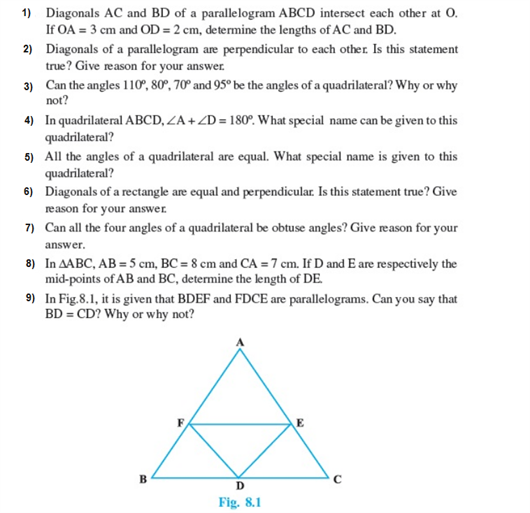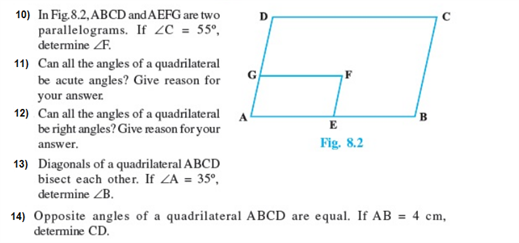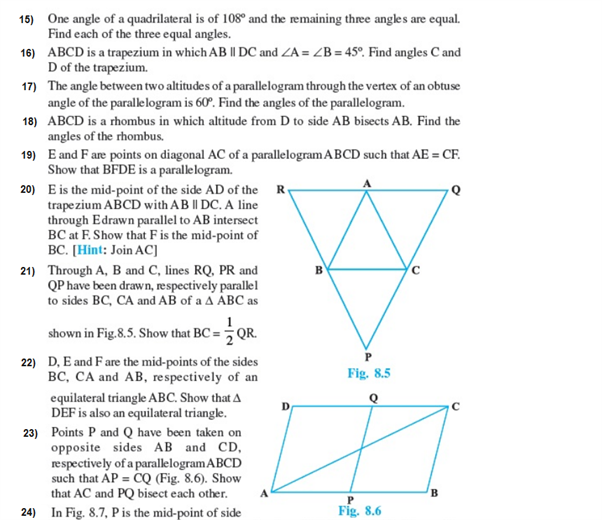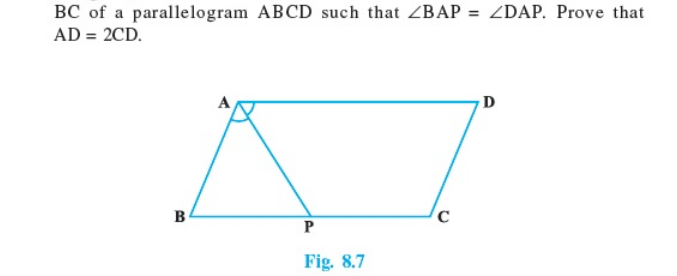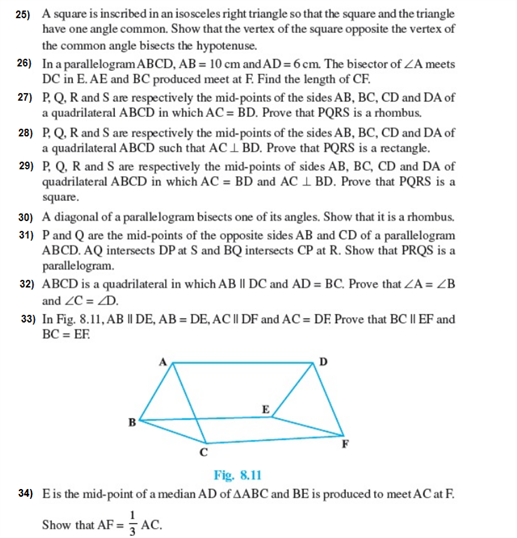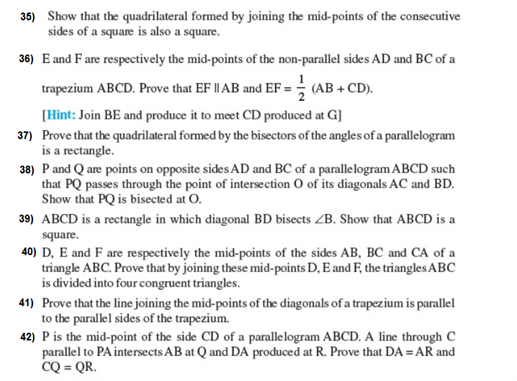# Important Questions Class 9 Maths Chapter 8 Quadrilaterals

Important class 9 Maths questions for chapter 8 Quadrilaterals provided here covers different question variations which will help the students to get completely acquainted with all the concepts. These CBSE class 9 questions for quadrilaterals include several short answer, long answer and HOTS type questions which will let the students develop their problem-solving skills.

Also Check:

## Important Quadrilateral Questions for Class 9- Chapter 8 (With Solutions)

Solution:

A quadrilateral is a 4 sided polygon having a closed shape. It is a 2-dimensional shape.

The 6 types of quadrilaterals include:

• Rectangle
• Square
• Parallelogram
• Rhombus
• Trapezium
• Kite

2. The diagonals of which quadrilateral are equal and bisect each other at 90°?

Solution:

Square. The diagonals of a square are equal and bisect each other at 90°.

3. Identify the type of quadrilaterals:

(i) The quadrilateral formed by joining the midpoints of consecutive sides of a quadrilateral whose diagonals are perpendicular.

(ii) The quadrilateral formed by joining the midpoints of consecutive sides of a quadrilateral whose diagonals are congruent.

Solution:

(i) The quadrilateral formed by joining the midpoints of consecutive sides of a quadrilateral whose diagonals are perpendicular is a rectangle.

(ii) The quadrilateral formed by joining the midpoints of consecutive sides of a quadrilateral whose diagonals are congruent is a rhombus.

4. Find all the angles of a parallelogram is one angle is 80°.

Solution:

For a parallelogram ABCD, opposite angles are equal.

So, the angles opposite to the given 80° angle will also be 80°.

It is also known that the sum of angles of any quadrilateral = 360°.

So, if ∠A = ∠C = 80° then,

∠A + ∠B + ∠C + ∠D = 360°

Also, ∠B = ∠C

Thus,

80° + B + 80° + D = 360°

Or, ∠B +∠ D = 200°

Hence, ∠B = ∠D = 100°

Now, all angles of the quadrilateral are found which are:

∠A = 80°

∠B = 100°

∠C = 80°

∠D = 100°

5. In a rectangle, 1 diagonal is inclined to one of its sides at 25°. Measure the acute angle between the two diagonals?

Solution:

Let ABCD be a rectangle where AC and BD are the two diagonals which are intersecting at point O.

Now, assume ∠BDC = 25° (given)

Now, ∠BDA = 90° – 25° = 65°

Also, ∠DAB = ∠BDA, (as diagonals of a rectangle divide the rectangle into two congruent right triangles)

So, ∠BOA = the acute angle between the two diagonals = 180° – 65° – 65°

6. Is it possible to draw a quadrilateral whose all angles are obtuse angles?

Solution:

It is known that the sum of angles of a quadrilateral is always 360°. To have all angles as obtuse, the angles of the quadrilateral will be greater than 360°. So, it is not possible to draw a quadrilateral whose all angles are obtuse angles.

7. Prove that the angle bisectors of a parallelogram form a rectangle.

Solution:

(Self-assessment)

8. In a trapezium ABCD, ABCD. Calculate C and D if A = 55° and B = 70°

Solution:

In a trapezium ABCD, ∠A + ∠D = 180° and ∠B + ∠C = 180°

So, 55° + ∠D = 180°

Or, ∠D = 125°

Similarly,

70° + ∠C = 180°

Or, ∠C = 110°

9. Calculate all the angles of a parallelogram if one of its angles is twice its adjacent angle.

Solution:

Let the angle of the parallelogram given in the question statement be “x”.

Now, its adjacent angle will be 2x.

It is known that the opposite angles of a parallelogram are equal.

So, all the angles of a parallelogram will be x, 2x, x, and 2x

As the sum of interior angles of a parallelogram = 360°,

x + 2x + x + 2x = 360°

Or, x = 60°

Thus, all the angles will be 60°, 120°, 60°, and 120°.

10. Calculate all the angles of a quadrilateral if they are in the ratio 2:5:4:1.

Solution:

As the angles are in the ratio 2:5:4:1, they can be written as-

2x, 5x, 4x, and x

Now, as the sum of the angles of a quadrilateral is 360°,

2x + 5x + 4x + x = 360°

Or, x = 30°

Now, all the angles will be,

2x =2 × 30° = 60°

5x = 5 × 30° = 150°

4x = 4 × 30° = 120°, and

x = 30°

### Extra Questions For Class 8 Chapter 10: Quadrilaterals (NCERT)